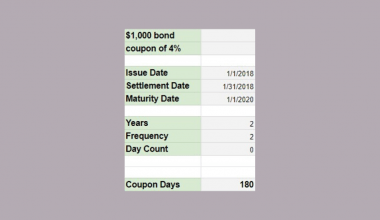# How To Use ISLOGICAL Function in Google Sheets

The ISLOGICAL function in Google Sheets is useful when you need to check if a given cell contains a boolean value.

Boolean values are denoted by either a ‘TRUE’ or ‘FALSE’ string, a checkbox, or a logical test output.

The rules for using the ISLOGICAL function in Google Sheets are as follows:

• The function requires a single cell with the value to verify as a boolean.
• The function then outputs TRUE or FALSE depending on whether the given value is a boolean.

Let’s take a look at a brief example!

In this example, we have a dataset of values that requires some data cleaning. Data cleaning is an important part of data analysis. This process involves detecting and correcting incomplete or inaccurate values in datasets. For example, rows with missing values can be filtered out or have those missing values filled with some specified value (like 0 or the mean of that given column).

Our dataset contains a column that requires every value to be a boolean. How do we make sure that each row in our dataset has a valid boolean value?

With the `ISLOGICAL` function it becomes quite easy to flag invalid boolean values. We can expand further on this function and perform specific operations on invalid values, or simply filter them out altogether.

Now that we know one common use-case of the `ISLOGICAL` function, let’s learn how to use it and work on an actual sample spreadsheet.

## The Anatomy of the ISLOGICAL Function

So the syntax (the way we write it) of the `ISLOGICAL` function is as follows:

`=ISLOGICAL(value)`

Let’s dissect this formula and understand what each of these terms means:

• = the equal sign is how we start any function in Google Sheets.
• ISLOGICAL() is our `ISLOGICAL` function. It returns whether the value of a given cell is `TRUE` or `FALSE`.
• value is the sole argument of the `ISLOGICAL` function. It may be a value, or a reference to another cell.
• The `ISLOGICAL` function is often used together with the `IF` function in conditional statements.

## A Real Example of Using ISLOGICAL Function

Let’s look into an example of the `ISLOGICAL` function being used in a Google Sheets spreadsheet.

In the example below, we have multiple types of values in Column A. If we only want to get values that correspond to either `TRUE` or `FALSE`, we can use the `ISLOGICAL` function.To get the values in Column B, we just need to use the following formula:

`=ISLOGICAL(A2)`

We can also use the `ISLOGICAL` function in Google Sheets in conjunction with other functions.

In the sample table below, we have a dataset where each row has a unique ID. Let’s say we would like to only see the IDs of rows with a valid boolean value. We can use the `FILTER` function with the `ISLOGICAL` function to return the list of valid IDs in column E.If you’re ready to test the `ISLOGICAL` function in Google Sheets, we can start writing it ourselves using the guide in the next section!

## How to Use ISLOGICAL Function in Google Sheets

1. First, select the cell we will be typing our formula in. In this example, we’ll start with cell B2.2. In our next step, we just have to type the equal sign ‘=‘ to begin our function, followed by ‘ISLOGICAL(‘.
3. A tooltip box may appear showing information on the `ISLOGICAL` function. We can click the arrow on the top-right-hand corner of the box to minimize it if needed.4. Next, we need to type in the value we’ll be verifying with the `ISLOGICAL` function. In this case, we can just use a reference to cell A2. Afterward, simply hit the Enter key on your keyboard to let the function evaluate.5. Finally, we can drag down the formula to fill out the rest of the column!1. How do I let the ISLOGICAL function return an integer value instead?
In case you need to return the result of the `ISLOGICAL` function as an integer, we can simply use the following modified formula:
=–ISLOGICAL(value)
2. How do I add a checkbox to my sheet?
Checkboxes are an easy way to make it easy for users to change the Boolean value of a cell. After selecting which cells we want to add checkboxes to, just click Insert and then click Checkbox to add one to all selected cells.This step-by-step guide shows how simple it is to determine whether a given value or cell has a Boolean value of TRUE or FALSE.

We can now use the ISLOGICAL functions in Google Sheets together with the various other Google Sheets formulas available to create great worksheet solutions.

Don’t miss staying up-to-date on our new guides by subscribing to our newsletterOur goal this year is to create lots of rich, bite-sized tutorials for Google Sheets users like you. If you liked this one, you'd love what we are working on! Readers receive ✨ early access ✨ to new content.

##### You May Also Like## How to Use the RATE Function in Google Sheets

The RATE function in Google Sheets is used to calculate the interest rate of an annuity investment based…## How to Use the SUMIF Function Horizontally in Google Sheets

The SUMIF function in Google Sheets is useful to get the sum of cells that meet the provided…## How to Use COUPDAYS Function in Google Sheets

Knowing how to use the COUPDAYS function in Google Sheets is useful if you want to calculate the…## How to Get Most Frequent Keywords from Titles in Google Sheets

There are a lot of functionalities that Google Sheets supports, like scraping data from websites, creating semi-automated SEO…

## How to Use BINOMDIST Function in Google Sheets

The BINOMDIST function in Google Sheets calculates for the binomial distribution or cumulative distribution of the probability. The…